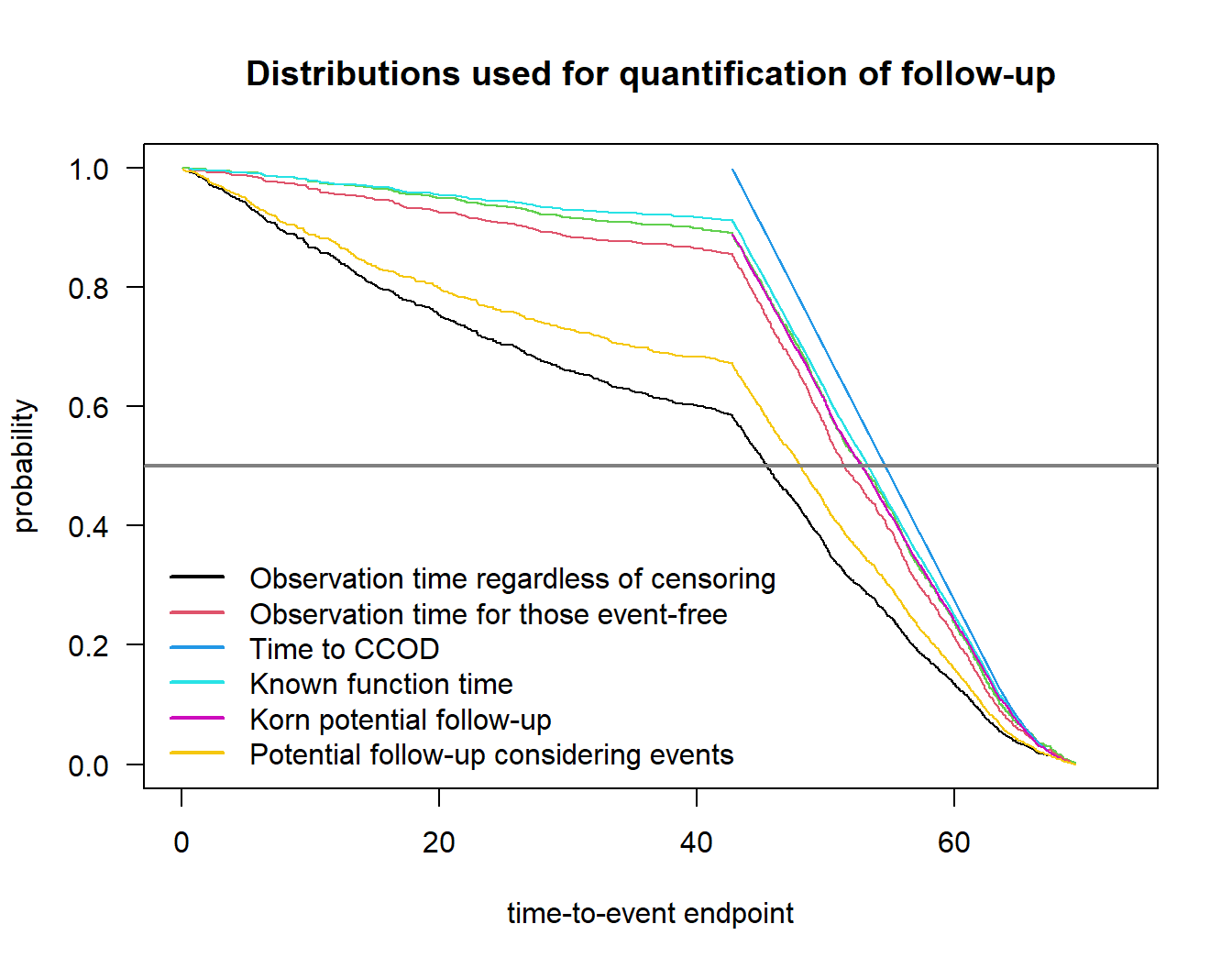# 1 Background

Code for the paper Rufibach et al. (2022) (download from arxiv) written by the “follow-up quantification” task force of the oncology estimand WG.

# 2 Purpose of this document

This R markdown file provides easy accessible code to compute all the quantifiers for follow-up. The github repository where this document is hosted is available here.

To illustrate the functions all clinical trial data in this file is simulated. In the paper, the PH examples uses real data.

# 3 Setup

## 3.1 Packages

# --------------------------------------------------------------
# packages
# --------------------------------------------------------------
packs <- c("survival", "rpact", "survRM2")
for (i in 1:length(packs)){library(packs[i], character.only = TRUE)}
## Warning: Paket 'rpact' wurde unter R Version 4.1.3 erstellt

## 3.2 Functions

Below all functions are defined.

• quantifyFU: This function provides all the seven methods described in the paper draft.
• plot.qfu: Plot the distributions from which medians are computed.
# --------------------------------------------------------------
# functions
# --------------------------------------------------------------

# function to compute all the different variants of follow-up quantification
quantifyFU <- function(rando, event_time, event_type, ccod){

## =================================================================
## compute all FU measures
## =================================================================

# input arguments:
# - rando: date of randomization
# - event_time: time to event or time to censoring
# - event_type: 0 = event, 1 = lost to FU, 2 = administratively censored
# - ccod: clinical cutoff date

require(survival)

n <- length(rando)

# objects to collect distributions
res <- NULL

# indicator for lost to follow up
ltfu_cens <- as.numeric(event_type == 1)

# usual censoring indicator: 0 = censored (for whatever reason), 1 = event
primary_event <- as.numeric(event_type == 0)

# indicator for censoring
event_time_cens <- as.numeric(primary_event == 0)

# observation time regardless of censoring
ecdf1 <- as.list(environment(ecdf(event_time)))
res[] <- cbind(ecdf1$x, 1 - ecdf1$y)
m1 <- median(event_time)

# observation time for those event-free
d2 <- event_time[event_time_cens == 1]
ecdf2 <- as.list(environment(ecdf(d2)))
res[] <- cbind(ecdf2$x, 1 - ecdf2$y)
m2 <- median(d2)

# time to censoring
so3 <- survfit(Surv(event_time, event_time_cens) ~ 1)
res[] <- so3
m3 <- summary(so3)$table["median"] # time to CCOD, potential followup pfu <- as.numeric((ccod - rando) / 365.25 * 12) ecdf4 <- as.list(environment(ecdf(pfu))) res[] <- cbind(ecdf4$x, 1 - ecdf4$y) m4 <- median(pfu) # known function time m5 <- rep(NA, n) m5[event_time_cens == 1] <- event_time[event_time_cens == 1] m5[primary_event == 1] <- pfu[primary_event == 1] ecdf5 <- as.list(environment(ecdf(m5))) res[] <- cbind(ecdf5$x, 1 - ecdf5$y) m5 <- median(m5) # Korn's potential follow-up time spfu <- sort(pfu) pt <- rep(NA, n) for (i in 1:n){ # timepoint at which we compute potential followup (t' in Schemper et al) t <- spfu[i] # proportion with pfu > t pet <- mean(spfu > t) # time to LTFU so6 <- survfit(Surv(event_time, ltfu_cens) ~ 1, subset = (pfu >= t)) pltet <- ifelse(max(so6$time > t) == 0, 0, so6$surv[so6$time > t])

pt[i] <- pltet * pet
}

res[] <- cbind(spfu, pt)

# now take the median of the distribution, see plot(t, pt, type = "s")
m6 <- max(spfu[pt >= 0.5])

# Potential follow-up considering events
m7 <- rep(NA, n)
m7[event_time_cens == 1] <- pfu[event_time_cens == 1]
m7[primary_event == 1] <- event_time[primary_event == 1]
ecdf7 <- as.list(environment(ecdf(m7)))
res[] <- cbind(ecdf7$x, 1 - ecdf7$y)
m7 <- median(m7)

# summarize results for medians
dat <- matrix(NA, nrow = 7, ncol = 1)
dat[, 1] <- c(m1, m2, m3, m4, m5, m6, m7)
rownames(dat) <- c("Observation time regardless of censoring", "Observation time for those event-free",
"Time to censoring", "Time to CCOD", "Known function time", "Korn potential follow-up",
"Potential follow-up considering events")
colnames(dat) <- "median"

output <- list("medians" = dat, "distributions" = res)
class(output) <- "qfu"
return(output)
}

# function to plot distributions of various quantifiers
plot.qfu <- function(x, which = 1:7, median = TRUE){

dat <- x$distributions par(las = 1) plot(0, 0, type = "n", xlim = c(0, 1.05 * max(dat[][, 1])), ylim = c(0, 1), xlab = "time-to-event endpoint", ylab = "probability", main = "Distributions used for quantification of follow-up") if (3 %in% which){lines(dat[], col = 3, conf.int = FALSE)} which <- which[which != 3] for (i in which){lines(dat[[i]], col = i)} if(isTRUE(median)){abline(h = 0.5, col = grey(0.5), lwd = 2)} legend("bottomleft", rownames(x$medians)[which], lty = 1, col = (1:7)[which], bty = "n", lwd = 2)
}

# compute value of KM estimate and CI
confIntKM_t0 <- function(time, event, t0, conf.level = 0.95){

alpha <- 1 - conf.level

# compute ci according to the formulas in Huesler and Zimmermann, Chapter 21
obj <- survfit(Surv(time, event) ~ 1, conf.int = 1 - alpha, conf.type = "plain", type = "kaplan", error = "greenwood", conf.lower = "peto")
St <- summary(obj)$surv t <- summary(obj)$time
n <- summary(obj)$n.risk res <- matrix(NA, nrow = length(t0), ncol = 3) for (i in 1:length(t0)){ ti <- t0[i] if (min(t) > ti){res[i, ] <- c(1, NA, NA)} if (min(t) <= ti){ if (ti %in% t){res[i, ] <- rep(NA, 3)} else { Sti <- min(St[t < ti]) nti <- min(n[t < ti]) Var.peto <- Sti ^ 2 * (1 - Sti) / nti Cti <- exp(qnorm(1 - alpha / 2) * sqrt(Var.peto) / (Sti ^ (3 / 2) * (1 - Sti))) ci.km <- c(Sti / ((1 - Cti) * Sti + Cti), Cti * Sti / ((Cti - 1) * Sti + 1)) res[i, ] <- c(Sti, ci.km)} } # end if } # end for res <- cbind(t0, res) dimnames(res)[] <- c("t0", "S at t0", "lower.ci", "upper.ci") return(res) } # bootstrap survival data bootSurvivalSample <- function(surv.obj, n, M = 1000, track = TRUE){ # bootstrap right-censored survival data according to Efron (1981) # see Akritas (1986) for details # surv.obj: Surv object to sample from # n: sample size for samples to be drawn # M: number of samples n.surv <- nrow(surv.obj) res.mat <- data.frame(matrix(NA, ncol = M, nrow = n)) for (i in 1:M){ ind <- sample(1:n.surv, size = n, replace = TRUE) res.mat[, i] <- surv.obj[ind] if (track){print(paste("sample ", i, " of ", M, " done", sep = ""))} } return(res.mat) } # compute value and CI for difference of survival probabilities at milestone, via bootstrap confIntMilestoneDiff <- function(time, event, group, t0, M = 10 ^ 3, conf.level = 0.95){ ind <- (group == levels(group)) s.obj1 <- Surv(time[ind], event[ind]) s.obj2 <- Surv(time[!ind], event[!ind]) n1 <- sum(ind) n2 <- sum(!ind) res1 <- bootSurvivalSample(surv.obj = s.obj1, n = n1, M = M, track = FALSE) res2 <- bootSurvivalSample(surv.obj = s.obj2, n = n2, M = M, track = FALSE) boot.diff1 <- rep(NA, M) boot.diff2 <- boot.diff1 for (i in 1:M){ # based on KM t1km <- confIntKM_t0(time = as.matrix(res1[, i])[, "time"], event = as.matrix(res1[, i])[, "status"], t0, conf.level = 0.95) t2km <- confIntKM_t0(time = as.matrix(res2[, i])[, "time"], event = as.matrix(res2[, i])[, "status"], t0, conf.level = 0.95) boot.diff1[i] <- t1km - t2km # based on exponential fit r1 <- exp(- coef(survreg(res1[, i] ~ 1, dist = "exponential"))) t1 <- 1 - pexp(t0, rate = r1) r2 <- exp(- coef(survreg(res2[, i] ~ 1, dist = "exponential"))) t2 <- 1 - pexp(t0, rate = r2) boot.diff2[i] <- t1 - t2 } # from bootstrap sample of differences just take symmetric quantiles to get CI for difference alpha <- 1 - conf.level ci1 <- quantile(boot.diff1, probs = c(alpha / 2, 1 - alpha / 2), na.rm = TRUE) ci2 <- quantile(boot.diff2, probs = c(alpha / 2, 1 - alpha / 2), na.rm = TRUE) res <- list("km" = ci1, "exponential" = ci2) return(res) } # compute the extreme limits as described by Betensky stabilityKM <- function(time, event){ ind <- (event == 0) maxevent <- max(time[event == 1]) # extreme scenarios (not precisely) according to Betensky (2015) # lower bound: every censored patient has event at censoring date t_low <- time c_low <- event c_low[ind] <- 1 # upper bound: every censored observation has censoring time equal to maximum event time t_up <- time t_up[ind & (time < maxevent)] <- maxevent c_up <- event # collect results res <- list("t_low" = t_low, "c_low" = c_low, "t_up" = t_up, "c_up" = c_up) return(res) } # 4 Example: Proportional hazards ## 4.1 Simulate a clinical trial with time-to-event endpoint using rpact A clinical trial with very similar properties as the Gallium data in Rufibach et al. (2022) is simulated using rpact Wassmer and Pahlke (2021). # simulate a clinical trial using rpact # time unit is months design <- getDesignGroupSequential(informationRates = 1, typeOfDesign = "asOF", sided = 1, alpha = 0.025, beta = 0.2) simulationResult <- getSimulationSurvival(design, lambda2 = log(2) / 60, hazardRatio = 0.75, dropoutRate1 = 0.025, dropoutRate2 = 0.025, dropoutTime = 12, accrualTime = 0:6, accrualIntensity = 6 * 1:7, plannedEvents = 350, directionUpper = FALSE, maxNumberOfSubjects = 1000, maxNumberOfIterations = 1, maxNumberOfRawDatasetsPerStage = 1, seed = 2021) # retrieve dataset simdat <- getRawData(simulationResult) # create variable with randomization dates # note that addition / subtraction of date objects happens in days # --> multiplication by 365.25 / 12 ~= 30 below day0 <- as.Date("2016-01-31", origin = "1899-12-30") rando <- day0 + simdat$accrualTime * 365.25 / 12
pfs <- simdat$timeUnderObservation # event type variable: 0 = event, 1 = lost to FU, 2 = administratively censored event_type <- rep(NA, nrow(simdat)) event_type[simdat$event == TRUE] <- 0
event_type[simdat$event == FALSE & simdat$dropoutEvent == TRUE] <- 1
event_type[simdat$event == FALSE & simdat$dropoutEvent == FALSE] <- 2

# PFS event
pfsevent <- as.numeric(event_type == 0)

# treatment arm
arm <- factor(simdat$treatmentGroup, levels = 1:2, labels = c("G", "R")) # define clinical cutoff date based on simulation result ccod <- day0 + simdat$observationTime * 365.25 / 12

# check
so1 <- summary(coxph(Surv(pfs, pfsevent) ~ arm))
so1
## Call:
## coxph(formula = Surv(pfs, pfsevent) ~ arm)
##
##   n= 1000, number of events= 350
##
##        coef exp(coef) se(coef)     z Pr(>|z|)
## armR 0.2351    1.2650   0.1073 2.191   0.0285 *
## ---
## Signif. codes:  0 '***' 0.001 '**' 0.01 '*' 0.05 '.' 0.1 ' ' 1
##
##      exp(coef) exp(-coef) lower .95 upper .95
## armR     1.265     0.7905     1.025     1.561
##
## Concordance= 0.528  (se = 0.014 )
## Likelihood ratio test= 4.82  on 1 df,   p=0.03
## Wald test            = 4.8  on 1 df,   p=0.03
## Score (logrank) test = 4.82  on 1 df,   p=0.03

Plot the Kaplan-Meier estimates:

par(las = 1)
plot(survfit(Surv(pfs, pfsevent) ~ arm), col = 2:3, mark = "'", lty = 1, xlim = c(0, 100),
xlab = "PFS", ylab = "probability of being event-free")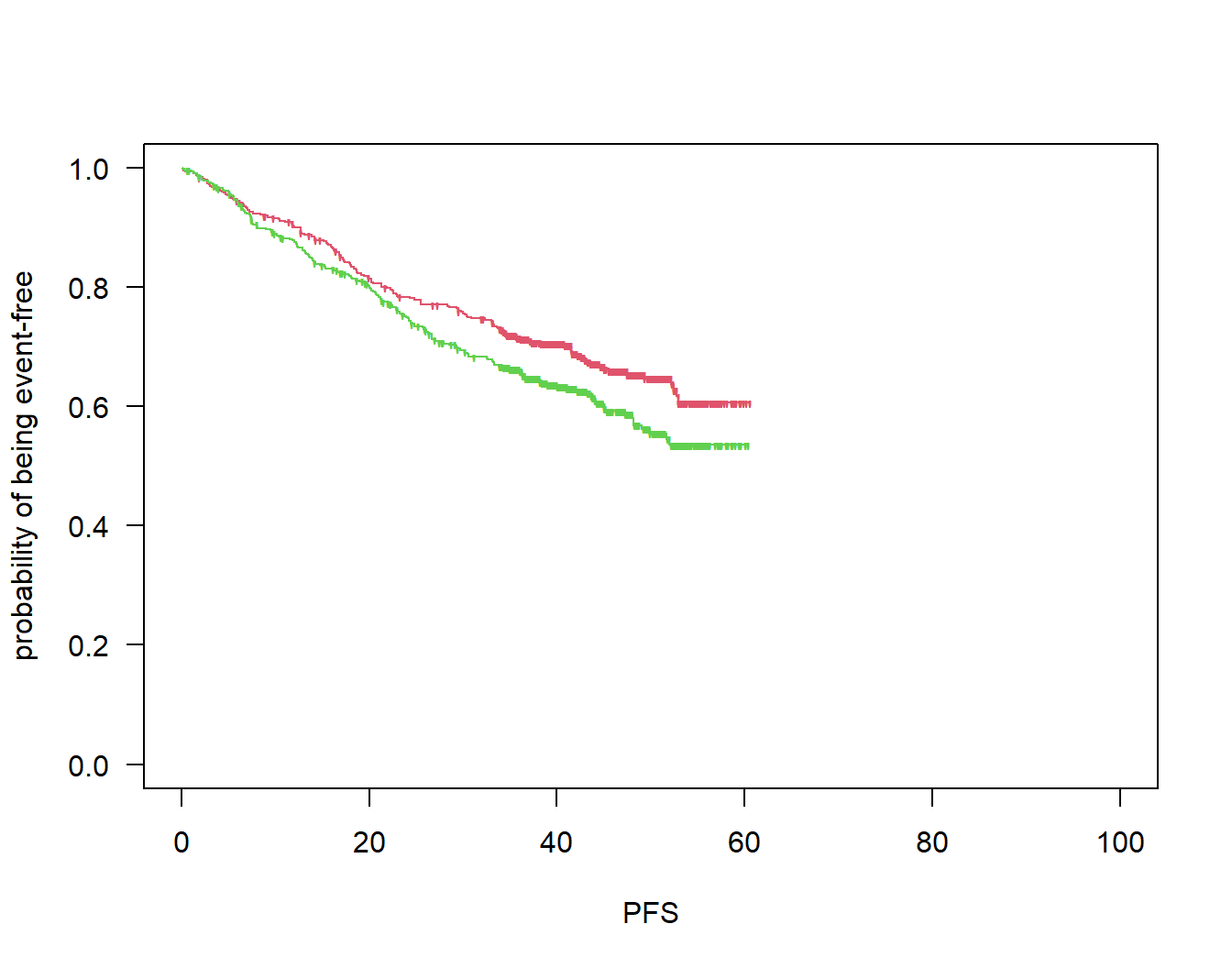## 4.2 Precision

Compute survival probabilities, their differences and confidence intervals at milestones.

ms <- c(36, 48)
msR1 <- confIntKM_t0(time = pfs[arm == "R"], event = pfsevent[arm == "R"], t0 = ms, conf.level = 0.95)
msG1 <- confIntKM_t0(time = pfs[arm == "G"], event = pfsevent[arm == "G"], t0 = ms, conf.level = 0.95)
msR1
##      t0   S at t0  lower.ci  upper.ci
## [1,] 36 0.6625629 0.6054758 0.7152741
## [2,] 48 0.5870704 0.4922056 0.6758830
msG1
##      t0   S at t0  lower.ci  upper.ci
## [1,] 36 0.7149897 0.6622073 0.7624827
## [2,] 48 0.6536542 0.5662833 0.7317616
# differences
ms1d1 <- confIntMilestoneDiff(time = pfs, event = pfsevent, group = arm,
t0 = ms, M = 10 ^ 3, conf.level = 0.95)$km ms2d1 <- confIntMilestoneDiff(time = pfs, event = pfsevent, group = arm, t0 = ms, M = 10 ^ 3, conf.level = 0.95)$km
ms1d1
##         2.5%        97.5%
## -0.003376164  0.114936362
ms2d1
##          2.5%         97.5%
## -0.0007250327  0.1350419043

## 4.3 Quantification of follow-up using various methods

Now let us apply the above functions:

fu <- quantifyFU(rando = rando, event_time = pfs, event_type = event_type, ccod = ccod)

# medians of all these distributions:
fu$medians ## median ## Observation time regardless of censoring 38.28363 ## Observation time for those event-free 42.87887 ## Time to censoring 44.05744 ## Time to CCOD 45.78363 ## Known function time 44.55744 ## Korn potential follow-up 44.15268 ## Potential follow-up considering events 40.31934 Plot distributions: plot(fu)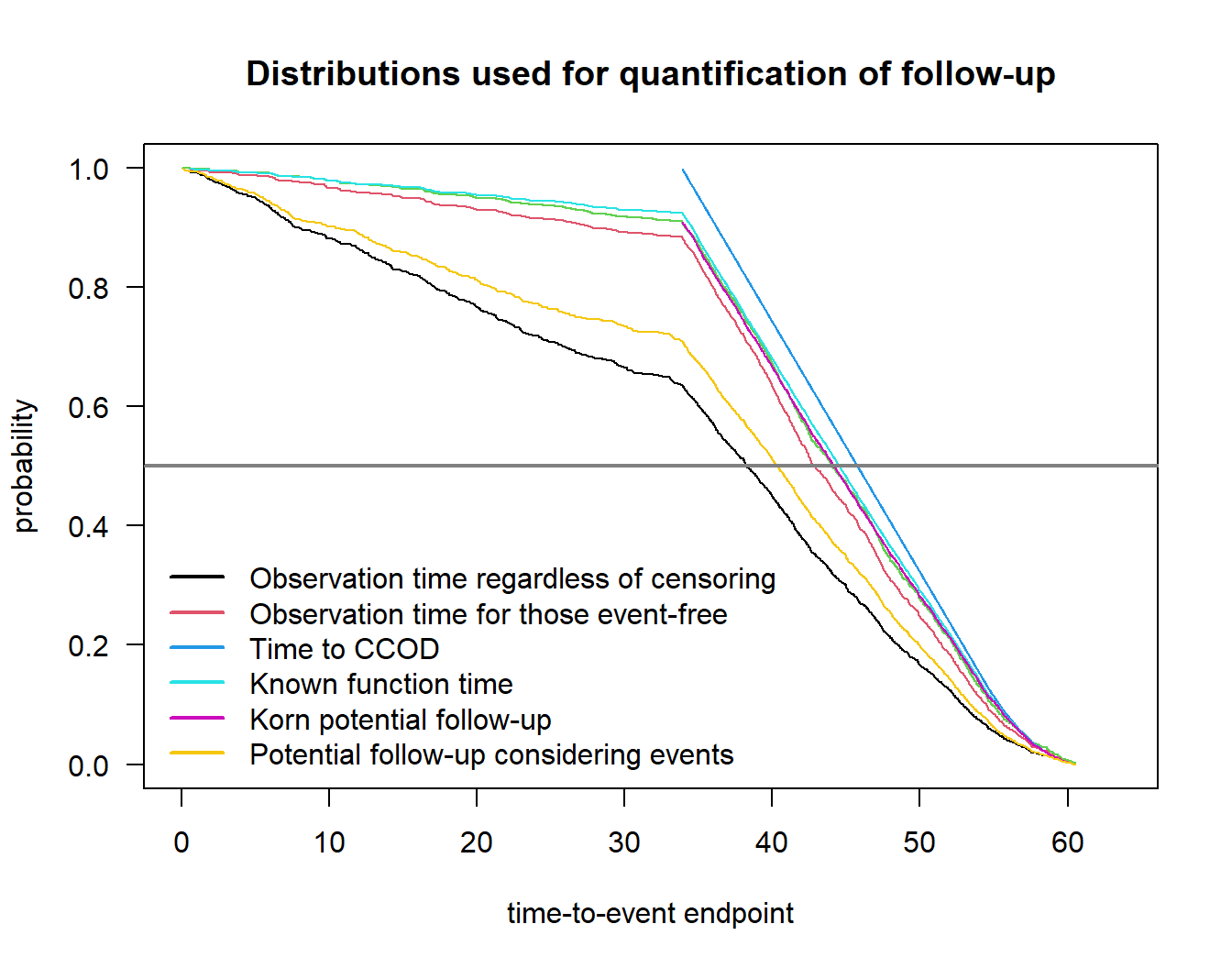# 5 Example: delayed separation ## 5.1 Simulate a clinical trial with time-to-event endpoint using rpact # Simulation assuming a delayed treatment effect alpha <- 0.05 beta <- 0.2 design <- getDesignGroupSequential(informationRates = 1, typeOfDesign = "asOF", sided = 1, alpha = alpha / 2, beta = beta) piecewiseSurvivalTime <- c(0, 12) baserate <- log(2) / 60 hr_nph <- 0.65 dropoutRate <- 0.025 dropoutTime <- 12 accrualTime <- 0:6 accrualIntensity <- 6 * 1:7 plannedEvents <- 389 maxNumberOfSubjects <- 1000 maxNumberOfIterations <- 10 ^ 3 simulationResultNPH <- getSimulationSurvival(design, piecewiseSurvivalTime = piecewiseSurvivalTime, lambda2 = c(baserate, baserate), lambda1 = c(baserate, hr_nph * baserate), dropoutRate1 = dropoutRate, dropoutRate2 = dropoutRate, dropoutTime = dropoutTime, accrualTime = accrualTime, accrualIntensity = accrualIntensity, plannedEvents = plannedEvents, directionUpper = FALSE, maxNumberOfSubjects = maxNumberOfSubjects, maxNumberOfIterations = maxNumberOfIterations, maxNumberOfRawDatasetsPerStage = 10 ^ 4, seed = 2021) # power for logrank test simulationResultNPH$overallReject
##  0.805
# access raw simulation data
simdat <- getRawData(simulationResultNPH)

# extract simulation run 1 for example in paper
simpick <- 1
simdat_run1 <- subset(simdat, iterationNumber == simpick)

# median time-to-dropout
med_do <- - log(2) / log((1- dropoutRate)) * dropoutTime

# create variable with randomization dates
# note that addition / subtraction of date objects happens in days
# --> multiplication by 365.25 / 12 ~= 30 below
day0_nph <- as.Date("2016-01-31", origin = "1899-12-30")
rando_nph <- day0_nph + simdat_run1$accrualTime * 365.25 / 12 pfs1_nph <- simdat_run1$timeUnderObservation

# event type variable: 0 = event, 1 = lost to FU, 2 = administratively censored
event_type_nph <- rep(NA, nrow(simdat_run1))
event_type_nph[simdat_run1$event == TRUE] <- 0 event_type_nph[simdat_run1$event == FALSE & simdat_run1$dropoutEvent == TRUE] <- 1 event_type_nph[simdat_run1$event == FALSE & simdat_run1$dropoutEvent == FALSE] <- 2 # PFS event pfsevent1_nph <- as.numeric(event_type_nph == 0) # treatment arm arm_nph <- factor(simdat_run1$treatmentGroup, levels = 1:2, labels = c("G", "R"))

# define clinical cutoff date based on simulation result
ccod1_nph <- day0_nph + simdat_run1$observationTime * 365.25 / 12 Plot Kaplan-Meier estimates: par(las = 1, mar = c(4.5, 4.5, 2, 1), oma = c(0, 0, 3, 0)) so1_nph <- survfit(Surv(pfs1_nph, pfsevent1_nph) ~ arm_nph) plot(so1_nph, col = 2:3, mark = "'", lty = 1, xlim = c(0, 100), xlab = "PFS (months)", ylab = "probability of not having a PFS event") abline(v = ms, col = grey(0.5), lwd = 2, lty = 2) # title mtext("Delayed separation", 3, line = 0, outer = TRUE)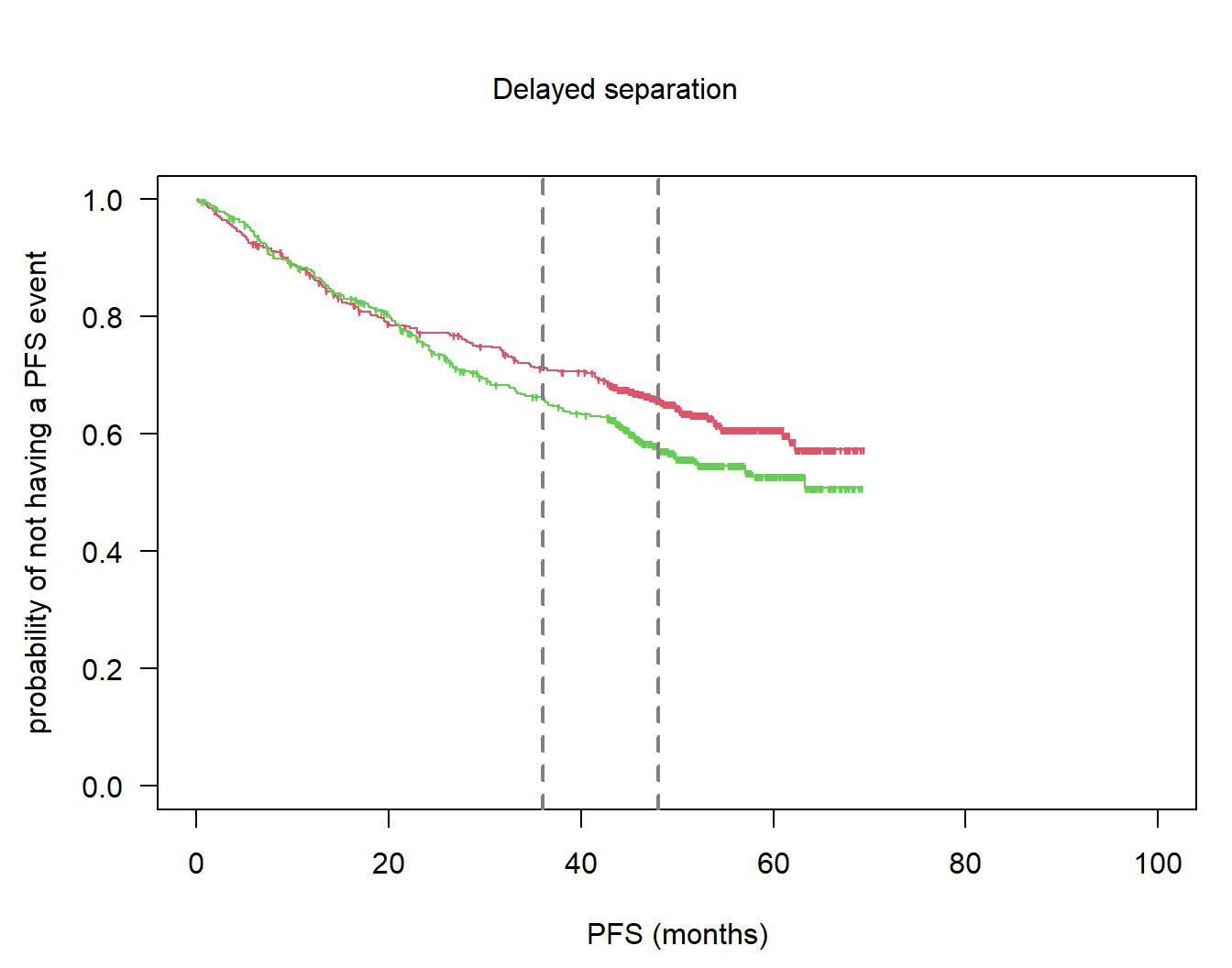## 5.2 Precision Compute survival probabilities, their differences and confidence intervals at milestones. ms <- c(36, 48) msR1_nph <- confIntKM_t0(time = pfs1_nph[arm == "R"], event = pfsevent1_nph[arm == "R"], t0 = ms, conf.level = 0.95) msG1_nph <- confIntKM_t0(time = pfs1_nph[arm == "G"], event = pfsevent1_nph[arm == "G"], t0 = ms, conf.level = 0.95) msR1_nph ## t0 S at t0 lower.ci upper.ci ## [1,] 36 0.6626743 0.6066027 0.7145152 ## [2,] 48 0.5801327 0.5109735 0.6462818 msG1_nph ## t0 S at t0 lower.ci upper.ci ## [1,] 36 0.7125514 0.6617708 0.7584903 ## [2,] 48 0.6571132 0.5948195 0.7144277 # differences ms1d1_nph <- confIntMilestoneDiff(time = pfs1_nph, event = pfsevent1_nph, group = arm, t0 = ms, M = 10 ^ 3, conf.level = 0.95)$km
ms2d1_nph <- confIntMilestoneDiff(time = pfs1_nph, event = pfsevent1_nph, group = arm,
t0 = ms, M = 10 ^ 3, conf.level = 0.95)$km ms1d1_nph ## 2.5% 97.5% ## -0.007217885 0.105350010 ms2d1_nph ## 2.5% 97.5% ## 0.01560682 0.13856650 ## 5.3 Stability par(las = 1, mar = c(4.5, 4.5, 2, 1), oma = c(0, 0, 3, 0)) so2_nph <- survfit(Surv(pfs1_nph, pfsevent1_nph) ~ 1, subset = (arm_nph == "G")) plot(so2_nph, col = 2, mark = "'", lty = 1, xlim = c(0, 100), xlab = "PFS (months)", ylab = "probability of not having a PFS event", conf.int = FALSE) abline(v = ms, col = grey(0.5), lwd = 2, lty = 2) # title mtext("Delayed separation", 3, line = 0, outer = TRUE) # add Betensky's scenarios stab_del_t <- stabilityKM(time = pfs1_nph[arm_nph == "G"], pfsevent1_nph[arm_nph == "G"]) stab_del_c <- stabilityKM(time = pfs1_nph[arm_nph == "R"], pfsevent1_nph[arm_nph == "R"]) # lower so2_nph_low <- survfit(Surv(stab_del_t$t_low, stab_del_t$c_low) ~ 1) lines(so2_nph_low, col = grey(0.5), lty = 1, conf.int = FALSE) # upper so2_nph_up <- survfit(Surv(stab_del_t$t_up, stab_del_t$c_up) ~ 1) lines(so2_nph_up, col = grey(0.5), lty = 1, conf.int = FALSE)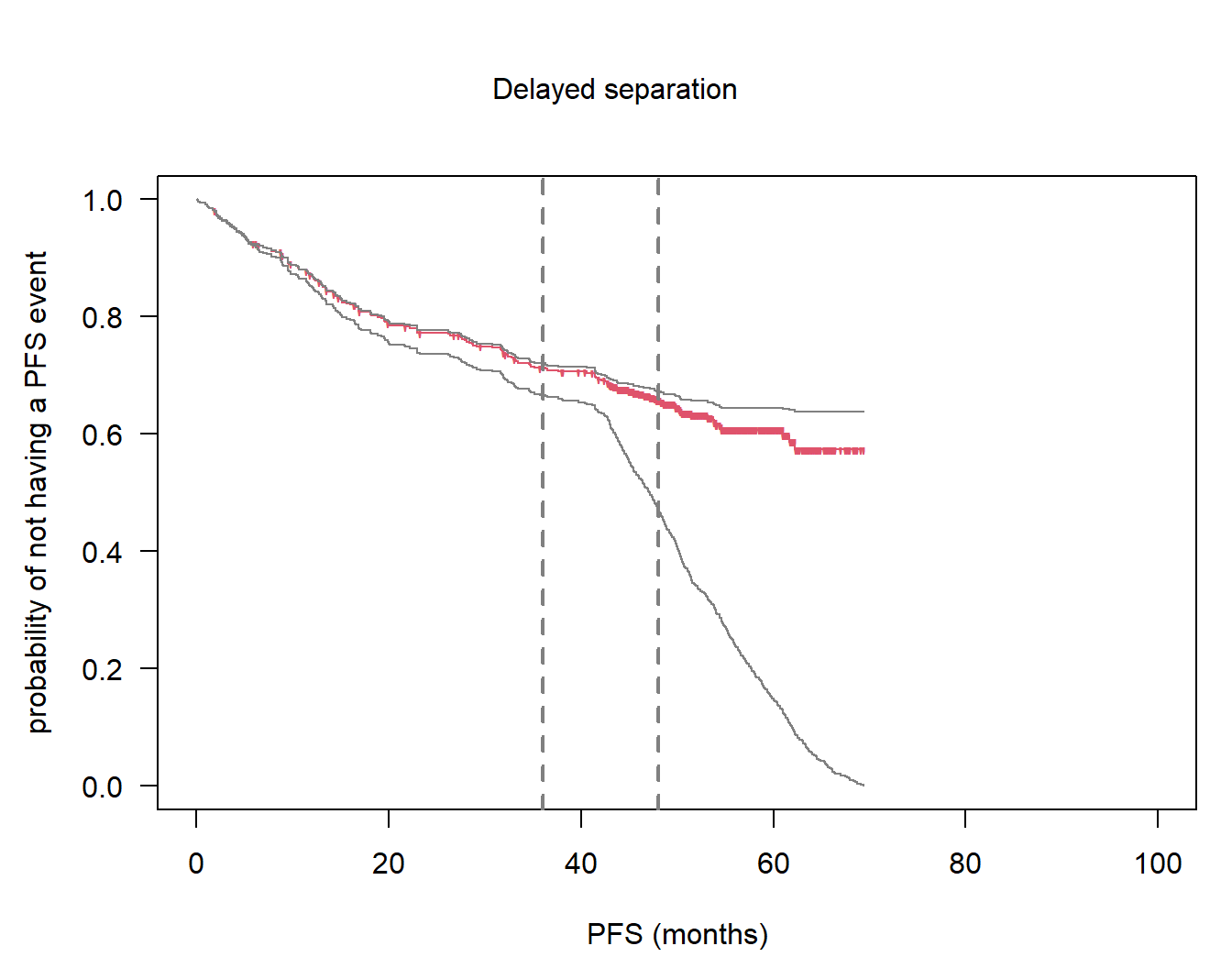## 5.4 Power for RMST # restriction timepoint for RMST tau <- NULL # use minimum of the two last observed times in each arm # compute RMST for every simulated dataset # use simulationResultNPH from p30 rmst_est <- rep(NA, maxNumberOfIterations) rmst_pval <- rmst_est logrank_pval <- rmst_est for (i in 1:maxNumberOfIterations){ sim.i <- subset(simdat, iterationNumber == i) time <- sim.i$timeUnderObservation
event <- as.numeric(sim.i$event) arm <- factor(as.numeric(sim.i$treatmentGroup == 1))

rmst <- rmst2(time = time, status = event, arm = arm, tau = NULL)
if (i == 1){rmst_sim1 <- rmst}

# we look at difference in RMST between arms
rmst_est[i] <- rmst$unadjusted.result[1, "Est."] rmst_pval[i] <- rmst$unadjusted.result[1, "p"]

# logrank p-value (score test from Cox regression)
logrank_pval[i] <- summary(coxph(Surv(time, event) ~ arm))$sctest["pvalue"] } # empirical power rmst_power <- mean(rmst_pval <= 0.05) rmst_power ##  0.692 mean(logrank_pval <= 0.05) ##  0.805 simulationResultNPH$overallReject
##  0.805

## 5.5 Quantification of follow-up using various methods

Now let us apply the above functions:

# compute various follow-up quantities
fu1_nph <- quantifyFU(rando = rando_nph, event_time = pfs1_nph,
event_type = event_type_nph, ccod = ccod1_nph)
fu1med_nph <- fu1_nph\$medians

# medians of all these distributions:
fu1med_nph
##                                            median
## Observation time regardless of censoring 45.47638
## Observation time for those event-free    51.46786
## Time to censoring                        52.84881
## Time to CCOD                             54.64643
## Known function time                      53.31310
## Korn potential follow-up                 52.80119
## Potential follow-up considering events   48.07205

Plot distributions:

plot(fu1_nph)